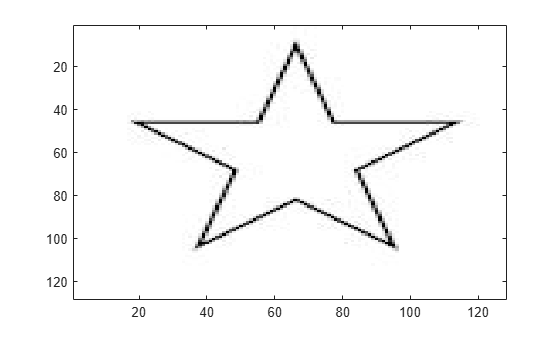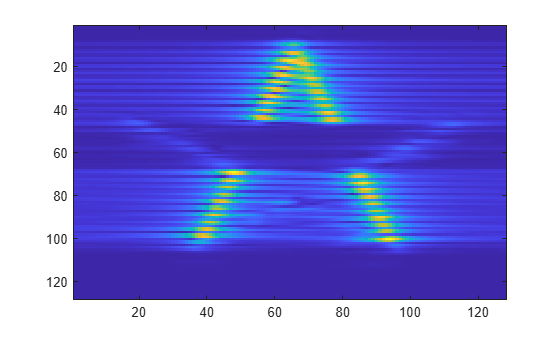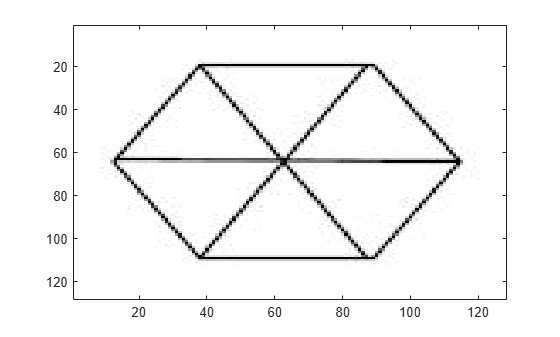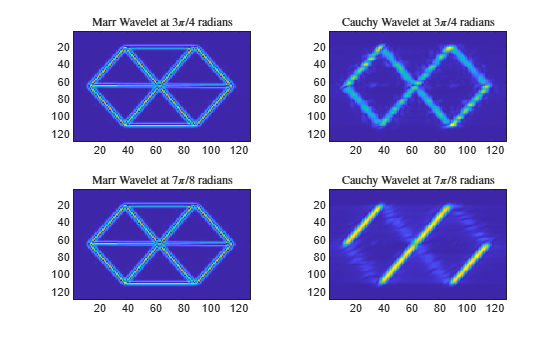# cwtft2

2-D continuous wavelet transform

## Syntax

``cwtstruct = cwtft2(X)``
``cwtstruct = cwtft2(X,"plot")``
``cwtstruct = cwtft2(___,Name,Value)``

## Description

example

````cwtstruct = cwtft2(X)` returns the 2-D continuous wavelet transform (CWT) of `X`.```

example

````cwtstruct = cwtft2(X,"plot")` plots the data and the 2-D CWT.```

example

````cwtstruct = cwtft2(___,Name,Value)` specifies options using one or more name-value arguments in addition to the input arguments in previous syntaxes.```

## Examples

collapse all

Load and display the star image.

```img = imread("star.jpg"); image(img)```Obtain the 2-D CWT of the star image using the default Morlet wavelet, scales `2.^(0:5)`, and an angle of 0. Visualize the 2-D CWT coefficient magnitudes at the finest scale.

```cwtout = cwtft2(img); sca = 1; imagesc(abs(cwtout.cfs(:,:,1,1,sca)))```Load an image of a woman, obtain the 2-D CWT using the default Morlet wavelet, and plot the CWT coefficients.

```load woman cwtmorl = cwtft2(X,"plot");```Shows how an isotropic wavelet does not discern the orientation of features while an anisotropic wavelet does. The example uses the Marr isotropic wavelet and the directional (anisotropic) Cauchy wavelet.

Load and view the hexagon image.

```img = imread("hexagon.jpg"); imagesc(img)```Obtain the scale-one 2-D CWT with both the Marr and Cauchy wavelets. Specify a vector of angles going from 0 to $15\pi /8$ in $\pi /8$ increments.

```cwtAngles = 0:pi/8:2*pi-pi/8; cwtcauchy = cwtft2(img,wavelet="cauchy",scales=1, ... angles=cwtAngles); cwtmarr = cwtft2(img,wavelet="marr",scales=1, ... angles=cwtAngles);```

There are 16 angles. Visualize the scale-one 2-D CWT coefficient magnitudes at any two consecutive angles. Confirm that using the Marr isotropic wavelet does not discern the orientation of features, but the Cauchy wavelet does.

```angz = {"0", "pi/8", "pi/4", "3pi/8", "pi/2", "5pi/8", "3pi/4", ... "7pi/8","pi", "9pi/8", "5pi/4", "11pi/8", "3pi/2", ... "13pi/8" "7pi/4", "15pi/8"}; indexAngle1 = 7; indexAngle2 = 8; tiledlayout(2,2) for k=[indexAngle1 indexAngle2] nexttile imagesc(abs(cwtmarr.cfs(:,:,1,1,k))); title(["Marr Wavelet at " angz(k) "radians"]); nexttile imagesc(abs(cwtcauchy.cfs(:,:,1,1,k))); title(["Cauchy Wavelet at " angz(k) "radians"]); end```Visualize the scale-one 2-D CWT coefficient magnitudes obtained using the Marr isotropic wavelet at any two angles. Confirm the wavelet does not discern the orientation of features.

```indexAngle1 = 2; indexAngle2 = 7; tiledlayout(1,2) for k=[indexAngle1 indexAngle2] nexttile imagesc(abs(cwtmarr.cfs(:,:,1,1,k))); title(["Marr Wavelet at " angz(k) "radians"]); end```## Input Arguments

collapse all

Input data, specified as a numeric array. `X` can be an M-by-N array representing an indexed image or an M-by-N-by-3 array representing a truecolor image.

Data Types: `double` | `single` | `uint8`

### Name-Value Arguments

Specify optional pairs of arguments as `Name1=Value1,...,NameN=ValueN`, where `Name` is the argument name and `Value` is the corresponding value. Name-value arguments must appear after other arguments, but the order of the pairs does not matter.

Example: `wavelet="paul",scales=2.^(0:5)` specifies to use the Paul wavelet and a vector of scales.

Before R2021a, use commas to separate each name and value, and enclose `Name` in quotes.

Example: `"wavelet","paul","scales",2.^(0:5)` specifies to use the Paul wavelet and a vector of scales.

Angles in radians used in the 2-D CWT, specified as a scalar or a vector.

Example: `angles=[0 pi/2 pi]`

Normalization used in the 2-D CWT, specified as one of these:

• `"L2"` — The Fourier transform of the analyzing wavelet at a given scale is multiplied by the corresponding scale. `"L2"` is the default normalization.

• `"L1"` — The Fourier transform of the analyzing wavelet is multiplied by 1 at all scales.

• `"L0"` — The Fourier transform of the analyzing wavelet at a given scale is multiplied by the square of the corresponding scale.

Example: `norm="L1"`

Scales, specified as a positive real-valued scalar or a vector of positive real numbers.

Example: `scales=2.^(1:6)`

Analyzing wavelet, specified as a character vector, a string scalar, a structure, or a cell array. `cwtftinfo2` provides a comprehensive list of supported wavelets and associated parameters.

If you specify `wavelet` as a structure, the structure must contain two fields:

• `name` — character vector or string scalar corresponding to a supported wavelet.

• `param` — cell array containing optional parameters, which depend on the wavelet. If you do not wish to specify optional parameters, use an empty cell array.

If you specify `wavelet` as a cell array, `wav`, the cell array must contain two elements:

• `wav{1}` — character vector or string scalar corresponding to a supported wavelet.

• `wav{2}` — cell array with the parameters of the wavelet.

Example: `"wavelet",{"morlet",{6,1,1}}` specifies the Morlet wavelet as a cell array.

Example: `"wavelet",struct("name","paul","param",{{2}})` specifies the Paul wavelet as a structure array.

## Output Arguments

collapse all

The 2-D CWT, returned as a structure with the following fields:

Analyzing wavelet and parameters, returned as a structure with the following fields:

• `wname` — Wavelet name

• `param` — Wavelet parameters

Normalization constants, returned as an M-by-N matrix, where M is the number of scales and N is the number of angles.

CWT coefficients, returned as an N-D array.

• The row and column dimensions of the array equal the row and column dimensions of the input data.

• The third page of the array is equal to 1 or 3 depending on whether the input data is a grayscale or truecolor image.

• The fourth page of the array is equal to the number of scales.

• The fifth page of the array is equal to the number of angles.

Scales for the 2-D CWT, returned as a row vector.

Angles for the 2-D CWT, returned as a row vector.

Mean of the input data, returned as a scalar

## Version History

Introduced in R2013b